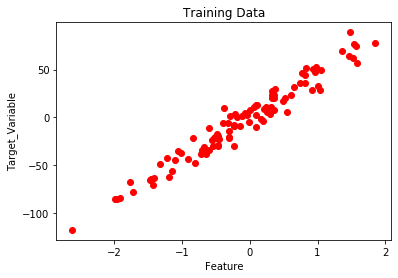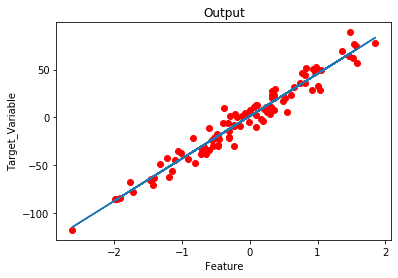In Machine Learning, Regression problems can be solved in the following ways:

1. Using Optimization Algorithms – Gradient Descent

• Other Advanced Optimization Algorithms like ( Conjugate Descent … )

2. Using the Normal Equation :

• Using the concept of Linear Algebra.

Let’s consider the case for Batch Gradient Descent for Univariate Linear Regression Problem.

The cost function for this Regression Problem is :Goal:In order to solve this problem, we can either go for a Vectorized approach ( Using the concept of Linear Algebra ) or unvectorized approach (Using for-loop).

### 1. Unvectorized Approach:

Here in order to solve the below mentioned mathematical expressions, We use for loop.

The above mathematical expression is a part of Cost Function.The above Mathematical Expression is the hypothesis.Code: Python Implementation of Unvectorzed Grad

 # Import required modules.  from sklearn.datasets import make_regression  import matplotlib.pyplot as plt  import numpy as np  import time      # Create and plot the data set.  x, y = make_regression(n_samples = 100, n_features = 1,                         n_informative = 1, noise = 10, random_state = 42)     plt.scatter(x, y, c = 'red')  plt.xlabel('Feature')  plt.ylabel('Target_Variable')  plt.title('Training Data')  plt.show()     # Convert y from 1d to 2d array.  y = y.reshape(100, 1)      # Number of Iterations for Gradient Descent  num_iter = 1000     # Learning Rate  alpha = 0.01     # Number of Training samples.  m = len(x)      # Initializing Theta.  theta = np.zeros((2, 1),dtype = float)      # Variables  t0 = t1 = 0 Grad0 = Grad1 = 0    # Batch Gradient Descent.  start_time = time.time()      for i in range(num_iter):      # To find Gradient 0.      for j in range(m):          Grad0 = Grad0 + (theta + theta * x[j]) - (y[j])             # To find Gradient 1.      for k in range(m):          Grad1 = Grad1 + ((theta + theta * x[k]) - (y[k])) * x[k]      t0 = theta - (alpha * (1/m) * Grad0)      t1 = theta - (alpha * (1/m) * Grad1)      theta = t0      theta = t1      Grad0 = Grad1 = 0         # Print the model parameters.      print('model parameters:',theta,sep = '\n')      # Print Time Take for Gradient Descent to Run.  print('Time Taken For Gradient Descent in Sec:',time.time()- start_time)     # Prediction on the same training set.  h = []  for i in range(m):      h.append(theta + theta * x[i])          # Plot the output.  plt.plot(x,h)  plt.scatter(x,y,c = 'red')  plt.xlabel('Feature')  plt.ylabel('Target_Variable')  plt.title('Output')

Output:model parameters:
[[ 1.15857049]
[44.42210912]]

Time Taken For Gradient Descent in Sec: 2.482538938522339### 2. Vectorized Approach:

Here in order to solve the below mentioned mathematical expressions, We use Matrix and Vectors (Linear Algebra).

The above mathematical expression is a part of Cost Function.The above Mathematical Expression is the hypothesis.#### Concept To Find Gradients  Using Matrix Operations:Code: Python implementation of vectorized Gradient Descent approach

 # Import required modules.  from sklearn.datasets import make_regression  import matplotlib.pyplot as plt  import numpy as np  import time      # Create and plot the data set.  x, y = make_regression(n_samples = 100, n_features = 1,                         n_informative = 1, noise = 10, random_state = 42)     plt.scatter(x, y, c = 'red')  plt.xlabel('Feature')  plt.ylabel('Target_Variable')  plt.title('Training Data')  plt.show()        # Adding x0=1 column to x array.  X_New = np.array([np.ones(len(x)), x.flatten()]).T     # Convert y from 1d to 2d array.  y = y.reshape(100, 1)      # Number of Iterations for Gradient Descent  num_iter = 1000     # Learning Rate  alpha = 0.01     # Number of Training samples.  m = len(x)      # Initializing Theta.  theta = np.zeros((2, 1),dtype = float)      # Batch-Gradient Descent.  start_time = time.time()      for i in range(num_iter):      gradients = X_New.T.dot(X_New.dot(theta)- y)      theta = theta - (1/m) * alpha * gradients      # Print the model parameters.      print('model parameters:',theta,sep = '\n')      # Print Time Take for Gradient Descent to Run.  print('Time Taken For Gradient Descent in Sec:',time.time() - start_time)     # Hypothesis.  h = X_New.dot(theta) # Prediction on training data itself.      # Plot the Output.  plt.scatter(x, y, c = 'red')  plt.plot(x ,h)  plt.xlabel('Feature')  plt.ylabel('Target_Variable')  plt.title('Output')

#### Output:model parameters:
[[ 1.15857049]
[44.42210912]]

Time Taken For Gradient Descent in Sec: 0.019551515579223633#### Observations:

1. Implementing a vectorized approach decreases the time taken for execution of Gradient Descent( Efficient Code ).
2. Easy to debug.

Attention geek! Strengthen your foundations with the Python Programming Foundation Course and learn the basics.

To begin with, your interview preparations Enhance your Data Structures concepts with the Python DS Course.

My Personal Notes arrow_drop_upCheck out this Author's contributed articles.

If you like GeeksforGeeks and would like to contribute, you can also write an article using contribute.geeksforgeeks.org or mail your article to contribute@geeksforgeeks.org. See your article appearing on the GeeksforGeeks main page and help other Geeks.

Please Improve this article if you find anything incorrect by clicking on the "Improve Article" button below.

Article Tags :
Practice Tags :

Be the First to upvote.

Please write to us at contribute@geeksforgeeks.org to report any issue with the above content.